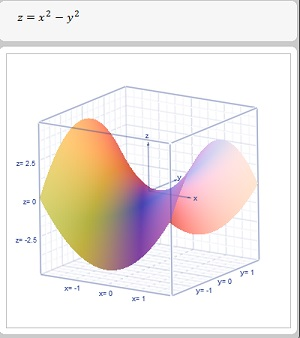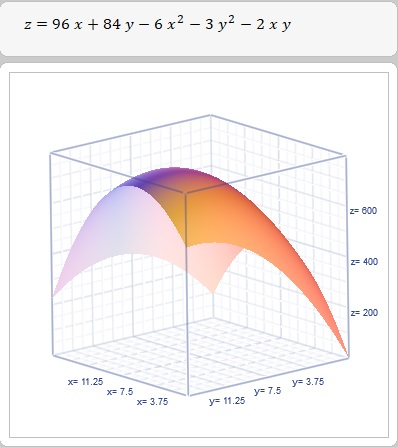# A firm produces and sells two commodities. When the firm produces x ton of the first commodity,...

## Question:

A firm produces and sells two commodities. When the firm produces {eq}x {/eq} ton of the first commodity, it is able to sell the commodity at a price of {eq}96 - 4x {/eq} dollars per ton. When the firm produces {eq}y {/eq} ton of the second commodity, it is able to sell the commodity at a price of {eq}84 - 2y {/eq} dollars per ton. The cost of producing and selling {eq}x {/eq} ton of the first commodity and {eq}y {/eq} ton of the second commodity is {eq}C(x,y) = 2x^{2} + 2xy + y^{2} {/eq}.

a) Find the firm's profit function {eq}\Pi(x,y) {/eq}.

b) Find all critical points of {eq}\Pi {/eq}. Classify each critical point as a local maximum, a local minimum, or a saddle point.

## Critical Points:

A function {eq}z = f(x,y) {/eq} attains a local maximum or a minimum or a saddle point at its critical points. A critical point is where the first order partial derivatives of the function either both vanish or not both exist. The former is when the function attains an optimum value - either a maximum or a minimum - or neither - which would be a saddle point.

Observe the graph below of a saddle point for the function, {eq}z = x^2 - y^2 {/eq}:We find the first order partial derivatives and the critical points of the above function below:

{eq}\frac{\delta z}{\delta x} = 2x = 0 \Longrightarrow x = 0 \\ \frac{\delta z}{\delta y} = -2y = 0 \Longrightarrow y = 0 \\ {/eq}

Note that the first order partial derivatives both vanish at {eq}(0,0) {/eq}. Hence this is a critical point for the function. However the function does not attain a maximum or a minimum, but has a saddle point here..

Hence the condition that the first order partial derivatives both vanish is not a sufficient condition to find a maxima or minima of a function. However if there were to occur an optimum value it is necessary that either both partial derivatives vanish or not both exist at the same point - which is the definition of a critical point for the function.

The cost function is given as

{eq}C(x,y) = 2x^{2} + 2xy + y^{2} {/eq}

The revenue function is computed below:

{eq}R(x,y) = x( 96 - 4x) + y ( 84 - 2y ) = 96x - 4x^2 + 84 y - 2y^2 {/eq}

a)The profit function would be,

{eq}\begin{align*} \Pi(x,y) &= R(x,y) - C(x, y) \\ &= 96x - 4x^2 + 84 y - 2y^2 - ( 2x^{2} + 2xy + y^{2}) \\ \Pi(x,y) &= 96x + 84y - 6x^2 - 3y^2 - 2xy \end{align*} {/eq}

The profit function is given by,

{eq}\displaystyle \boxed{ \Longrightarrow \Pi(x,y) = 96x + 84y - 6x^2 - 3y^2 - 2xy } {/eq}

b)The critical points of a function are where the first order partial derivatives of the function both vanish, or not both of them exist.

When both partial derivatives vanish we have a local maximum or a local minimum value attained by the function or a saddle point. The saddle point happens if, when viewed from one direction, the function has a maximum, but when viewed from another direction, the function appears to have a minimum, which is the general shape of a saddle. In this case, we have neither a maximum or a minimum at the point.

We find the critical points of the function as follows:

{eq}\begin{align*} \Pi(x,y) &= 96x + 84y - 6x^2 - 3y^2 - 2xy \\ \frac{\delta \Pi(x,y)}{\delta x} &= 96 -12x - 2y = 0 \\ \frac{\delta \Pi(x,y)}{\delta y} &=84 - 6y - 2x = 0 \end{align*} {/eq}

We solve the above simultaneous equations to find any critical points or saddle points for the function:

{eq}\begin{align*} 12x + 2y &= 96 \; &&\text{(1)} \\ 2x + 6y &= 84 \; &&\text{(2)} \\ 36x + 6y &= 288 \; &&\text{ 3 x (1)} \\ 34x &= 204 \; &&\text{3 x (1) - (2)} \\ x &= \frac{204}{34} \\ x &= 6 \\ 6y &= 84 - 2x \; &&\text{(2)} \\ 6y &= 84 - 2 \times 6 \\ 6y &= 84 - 12 \\ y &= \frac{72}{6} \\ y &= 12 \end{align*} {/eq}

The function has a critical point at {eq}( 6, 12) {/eq}.

There are no other critical points. The above point can be a maximum or a minimum. There are no given boundaries for the function. We can assume we want production of both commodities, or that at least one of the commodities be produced.

This gives us the boundary points, {eq}x = 0, \; y \neq 0, \; y = 0, x \neq 0 {/eq}

Observe the graph of the profit function shown below:The graph shows the function attains a local and absolute maximum at the critical point {eq}( 6, 12) {/eq}.

We conclude that there is only one critical point and that its a local maximum.